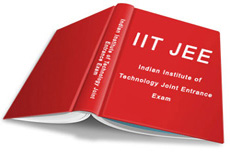#### Free Online Books

##### H C Verma’s Concepts of Physics Part 1 and Part 2

Considered a must work out assignment by most of the IIT aspirants.. Here you can find the solutions to the problems chapterwise...

##### I E Irodov - Problems in General Physics

Try solving these problems on your own first and use these solutions for verification...##### IIT JEE Past Year Papers

Links to various websites for Past Year Papers of IIT JEE with Solutions.

##### Top 100 Engineering Colleges

Here is the list of top 100 engineering colleges in India which will give you an idea about the best engineering colleges in India.

Home Tutor Networks : Tutors for any subject and any board (ICSE, IGCSE, IB, CBSE) starting from Nursery level to XII.

##### Physics : Part - I

General: Units and dimensions, dimensional analysis; least count, significant figures; Methods of measurement and error analysis for physical quantities pertaining to the following experiments: Experiments based on using Vernier calipers and screw gauge (micrometer), Determination of g using simple pendulum, Young’s modulus by Searle’s method, Specific heat of a liquid using calorimeter, focal length of a concave mirror and a convex lens using u-v method, Speed of sound using resonance column, Verification of Ohm’s law using voltmeter and ammeter, and specific resistance of the material of a wire using meter bridge and post office box.

Mechanics: Kinematics in one and two dimensions (Cartesian coordinates only), projectiles; Uniform Circular motion; Relative velocity.

Newton’s laws of motion; Inertial and uniformly accelerated frames of reference; Static and dynamic friction; Kinetic and potential energy; Work and power; Conservation of linear momentum and mechanical energy.

Systems of particles; Centre of mass and its motion; Impulse; Elastic and inelastic collisions.

Law of gravitation; Gravitational potential and field; Acceleration due to gravity; Motion of planets and satellites in circular orbits; Escape velocity.

Rigid body, moment of inertia, parallel and perpendicular axes theorems, moment of inertia of uniform bodies with simple geometrical shapes; Angular momentum; Torque; Conservation of angular momentum; Dynamics of rigid bodies with fixed axis of rotation; Rolling without slipping of rings, cylinders and spheres; Equilibrium of rigid bodies; Collision of point masses with rigid bodies.

Linear and angular simple harmonic motions.

Hooke’s law, Young’s modulus.

Pressure in a fluid; Pascal’s law; Buoyancy; Surface energy and surface tension, capillary rise; Viscosity (Poiseuille’s equation excluded), Stoke’s law; Terminal velocity, Streamline flow, equation of continuity, Bernoulli’s theorem and its applications.

Wave motion (plane waves only), longitudinal and transverse waves, superposition of waves; Progressive and stationary waves; Vibration of strings and air columns;Resonance; Beats; Speed of sound in gases; Doppler effect (in sound).

Thermal physics: Thermal expansion of solids, liquids and gases; Calorimetry, latent heat; Heat conduction in one dimension; Elementary concepts of convection and radiation; Newton’s law of cooling; Ideal gas laws; Specific heats (Cv and Cp for monoatomic and diatomic gases); Isothermal and adiabatic processes, bulk modulus of gases; Equivalence of heat and work; First law of thermodynamics and its applications (only for ideal gases);  Blackbody radiation: absorptive and emissive powers; Kirchhoff’s law; Wien’s displacement law, Stefan’s law.